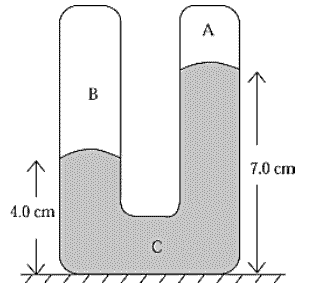# Manometer U Tube

Homework StatementThe U-tube in the figure is sealed at both ends. It contains a gas in A, another gas in B, and mercury in C. The heights of the mercury in the two arms are as shown. The density of mercury is 13.6 g/cm3. If the pressure in A is 1 × 10^4 dyn/cm2, the pressure in B is

The attempt at a solution

4-7 = 3CM

0.03m * 9.81 * 13600 kg/m^3 which = 4000 Pa which converts to 4x10^4 dyn/cm^2

But the answer given was 5x10^4 dyn/cm^2

I am not sure what I am doing wrong?

Edit: 4x10E4 is the pressure difference. So I have to add A which is 1x10E4 and that gives B = 5x10E4.

Could someone explain why I add A?

Last edited: